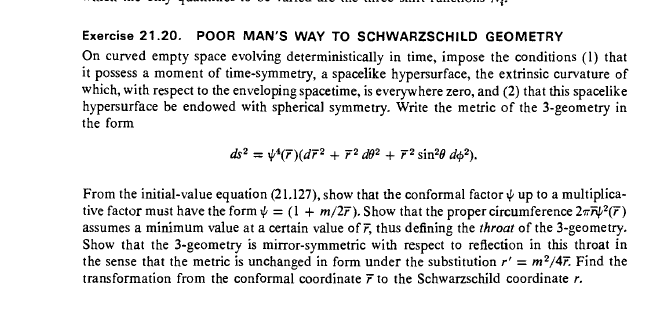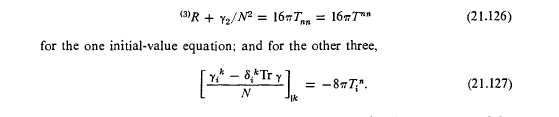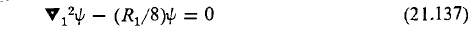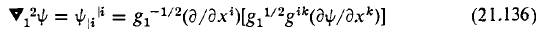# A poor man's way to Schwarzschild Geometry

Gold Member
Homework Statement:
The problem is MTW Exercise 21.10 (attached)
Relevant Equations:
The relevant equation is the Initial Value equation 21.127 (attached)
Can anyone help me get started with this problem?

What should I use for Gni?

I've tried to produce Tni by working out Rni (using methods developed in an earlier chapter) but the results don't lead me anywhere.

I'm really stuck for a way forward on this problem so if anyone can help, it would be much appreciated.

TerryWIbix
2020 Award
You should be able to write down ##T## by observation.

Hint: read the first line of the question.

Gold Member
Hi Ibix,

Thanks for coming to my assistance.

I started off by assuming that the first line of the question is telling me that ##K_{ij}= 0##. In which case,
(21.127) reduces to ##8\pi T_i{}^n =0##.

If ##T^{nn} = \rho## (which seems to be the implication of (21.132)) and ##T_i{}^n =g_{in}T^{nn}##

then I end up with ## g_{in} \rho = 0## or ##N_i\rho =0 ## if ##g_{in} = N_i## which doesn't help me very much.

I am clearly not seeing something here!!

Regards

TerryW

Ibix
2020 Award
I think that (1) tells you that ##K_{ij}=0##, yes. But before that it says "empty space", which tells you that the stress-energy tensor is zero. So I think you are being asked to solve the left hand side of 21.127 equals zero. That presumably nets you an expression for ##\psi##.

Gold Member
The left hand side of 21.127 is ##\frac{\gamma _i{}^k - \delta _i{}^k Tr\gamma}{N}##

If ##\gamma _{ij} = \frac {1}{2}(N_{i|j} +N_{j|i} - \frac{∂g_{ij}}{∂t}) = NK_{ij} ## and ##K_{ij} = 0##

then surely the LHS = 0 doesn't it?

TerryW

Ibix
2020 Award
Hm.

I think I'm out of my depth here - I'll send up the bat signal.

•jedishrfu
PeterDonis
Mentor
2020 Award
If ##\gamma _{ij} = \frac {1}{2}(N_{i|j} +N_{j|i} - \frac{∂g_{ij}}{∂t}) = NK_{ij} ##

Where are you getting the ##= N K_{ij}## part from?

PeterDonis
Mentor
2020 Award
then surely the LHS = 0 doesn't it?

It is true that the LHS of equation 21.127 has to be zero, because the RHS is zero (since the stress-energy tensor is zero). But that does not mean ##\gamma_{ij} = 0## or that the solution of equation 21.127 gives no information.

Gold Member
Hi Peter,

I was on Chapter 8 when you last came to my aid!
Where are you getting the ##= N K_{ij}## part from?

This comes straight from equation (21.67)

Regards

TerryW

PeterDonis
Mentor
2020 Award
This comes straight from equation (21.67)

Ok. But notice that equation 21.67 is also a differential equation, and saying that the LHS of 21.127 is zero (either because the RHS, the stress-energy tensor, is zero, or because ##K_{ij}## is zero) is just saying that the differential equation is homogeneous. In other words, you still have to solve the differential equation.

Gold Member
Ok, I've processed the differentiation as follows (in detail):

##\big( \frac{\gamma_i{}^k - \delta _i{}^k Tr \gamma}{N}\big)_{|k} = 0##

##-\frac{N_{|k}}{N^2}(\gamma_i{}^k - \delta _i{}^k Tr \gamma) +-\frac{1}{N}(\gamma_i{}^k - \delta _i{}^k Tr\gamma)_{|k} = 0##

The first term disappears because ##\gamma_{ij} = 0## leaving me to process:

##(\gamma_i{}^k - \delta _i{}^k Tr\gamma)_{|k} = 0##
##(g^{jk}\gamma_{ij|k} - \delta _i{}^k g^{lm} \gamma_{lm|k} = 0##
##g^{jk}(\gamma_{ij,k} -\Gamma^l{}_{ik}\gamma _{jl}-\Gamma^l{}_{jk}\gamma _{il}) - \delta _i{}^k g^{lm} (\gamma_{lm,k}-\Gamma^p{}_{lk}\gamma _{mp}-\Gamma^p{}_{mk}\gamma_{lp}) = 0##

All the terms with ##\Gamma## disappear because ##\gamma_{ij} = 0## leaving me with:

##g^{jk}\gamma_{ij,k} - g^{lm} \gamma_{lm,i} = 0##

Given that ##\gamma_{ij} = 0## everywhere on the hypersurface, then ##\gamma_{ij,k} = 0## everywhere too, leaving me with the unarguable 0 - 0 = 0, but nowhere near the answer!

Regards

TerryW

PeterDonis
Mentor
2020 Award
Given that ##\gamma_{ij} = 0## everywhere on the hypersurface, then ##\gamma_{ij,k} = 0## everywhere too

Not necessarily. The derivatives of ##\gamma_{ij}## involve the second derivatives of the lapse and shift, which are not necessarily zero on the hypersurface. The equation ##\gamma_{ij} = 0## is itself a differential equation.

Last edited:
PeterDonis
Mentor
2020 Award

A suggestion: the problem gives you the 3-metric. Try simply turning the crank: write down the lapse and shift functions and their derivatives, and the time derivative of the metric, which gives you an explicit formula for ##\gamma_{ij}##, then simply plug in to equation 21.127 and see what you get.

The discussion at the start of section 21.10 might also be helpful. (Note that that discussion seems to indicate that you might also want to look at equation 21.126, of which equation 21.132 is a simplified form.)

PeterDonis
Mentor
2020 Award
The discussion at the start of section 21.10 might also be helpful. (Note that that discussion seems to indicate that you might also want to look at equation 21.126, of which equation 21.132 is a simplified form.)

On going back and reworking this problem for myself again (instead of relying on memory), I think the above observation is key. I think the problem statement is misleading in mentioning only equation 21.127 (it's possible that that was a typo and that the intent was to direct attention at both 21.126 and 21.127).

Gold Member
Hi Peter,

Thanks for this. I'll go and have a think.

Regards

Terry W

Gold Member
Hi Peter,

I'm still not quite getting this!

I've explored the use of the formula:

##N_{i,j} + N_{j,i} - \frac{\partial g_{ij}}{\partial t} = N^p(g_{pi,j} +g_{pj,i} -g_{ij,p} )## derived from 21.67

Using the three components of the metric ##g_{rr}##, ##g_{\theta \theta}## and ##g_{\phi \phi}##
to produce expressions for ##N_{r,r}, N_{\theta,\theta}## and ##(N_{\theta,r} + N_{r,\theta})##
from which I was able to produce a differential equation for ##\psi##, but it was not a pretty sight and ##\psi = (1 + \frac{m}{2r})## clearly isn't a solution!

I also cannot see what I could possibly get out of 21.126 given that ##\gamma_{ij}## and ##\rho = 0##

What am I missing?

Regards

TerryW

PeterDonis
Mentor
2020 Award
the formula:

##N_{i,j} + N_{j,i} - \frac{\partial g_{ij}}{\partial t} = N^p(g_{pi,j} +g_{pj,i} -g_{ij,p} )## derived from 21.67

I don't see how your formula is equivalent to 21.67. Remember that ##t## in 21.67 is not a coordinate, it's a parameter. The indexes ##i, j## do not include ##t##.

Also, I believe the lapse ##N## and the shift ##N^i## can be chosen on the given hypersurface such that ##N = 1## and ##N^i = 0##. So 21.67 can be reduced to

##K_{ij} = - \frac{d g_{ij}}{dt}##

But you still need to figure out ##d g_{ij} / dt##, and there is no information given in the problem about how anything varies with ##t##. So I'm not sure this path leads anywhere, which is why I said I think the problem statement is misleading in only mentioning equation 21.127 and not 21.126.

I also cannot see what I could possibly get out of 21.126

21.126 for this case says that ##R = 0##, i.e, the Ricci curvature scalar for the 3-metric on the given hypersurface is zero. That gives you a differential equation for ##\psi##.

Last edited:
TSny
Homework Helper
Gold Member
I have not digested much of Chapter 21. But, it appears to me that equation (21.137) might be the easiest way to show that ##\psi( r)## in Exercise 21.20 has the form ## \left (1+ \large \frac{m}{2 r}\right)## up to an overall constant factor. The discussion on page 535 that leads up to (21.137) seems very relevant to the exercise. However, equation (21.137) comes from equation (21.135), and, as far as I can tell, equation (21.135) is not derived in the text. Instead, a reference is made to the old book of Eisenhart, Riemannian Geometry.

Gold Member
Hi Peter and TSny,

I don't see how your formula is equivalent to 21.67. Remember that t in 21.67 is not a coordinate, it's a parameter. The indexes i,j do not include t.

All I've done here is put ##K_{ik} = 0 ## and ##\Gamma_{pik}## with ##\frac{1}{2}(g_{pi,k} + g_{pk,i} - g_{ki,p})##

21.126 for this case says that R=0, i.e, the Ricci curvature scalar for the 3-metric on the given hypersurface is zero. That gives you a differential equation for ψ.
I think I'll go with this idea for the moment. (Using 21.137 looks more complicated!) - I can see that quite a bit of work is going to be required and I won't be around for the next few days, so it will be a while before I let you know how I've got on.

In the meantime, thanks for all the help.

Regards

TerryW

TSny
Homework Helper
Gold Member
In equation (21.137), ##R_1 = 0## for exercise 21.20. So, you're left with solving ##\mathbf \nabla_1^2 \psi = 0##.Use (21.136) where ##g_1## and ##g^{ik}## refer to the metric ##d \overline r^2 +\overline r^2 d\theta^2 + \overline r^2 \sin^2 \theta \, d \phi^2##.Last edited:
PeroK
Homework Helper
Gold Member
2020 Award
I think I'm out of my depth here - I'll send up the bat signal.
You're still in a different intellectual league from Chief O'Hara and Commissioner Gordon!

•Ibix
PeterDonis
Mentor
2020 Award
All I've done here is put ##K_{ik} = 0 ## and ##\Gamma_{pik}## with ##\frac{1}{2}(g_{pi,k} + g_{pk,i} - g_{ki,p})##

It is not the case that ##d g_{ik} / dt = N^p g_{ik,p}##. The contraction index ##p## cannot range over ##t##, because ##t## is not a coordinate in this formalism, and the index on ##N^p## cannot range over ##t##; ##t## is a parameter.

Gold Member
Hi Peter and TSny

FollowingTSny's suggestion of using 21.137 and 21.136, I've produced the required result, so thanks for that!

I'm still going to try to get the result by working out R in terms of derivatives of ##\psi## just for the satisfaction of seeing it all come out.

One question arising from all this - In obtaining the result, I ended up with an expression with a ratio of arbitrary constants. I can quite legitimately resolve these into ##\frac {m}{2}##, but what is ##m##?

Given that the problem statement defined an empty space, how can ##m## be a mass and if it isn't a mass, what exactly is it?

It is not the case that dgik/dt=Npgik,p. The contraction index p cannot range over t, because t is not a coordinate in this formalism, and the index on Np cannot range over t; t is a parameter.

Isn't it the case that t is a time parameter and that both N and N_p are functions of t and the space co-ordinates? Sure the summation can only take place over the three space co-ordinates, but if you move from one hyperplane to another, ##g_{ik}## will change and the space co-ordinates of the landing point on the later hyperplane will be different. Equations 21.36 and 21.27 and the whole introduction to ADM on pages 506 and 507 seem to point in this direction.

Regards

TerryW

Last edited:
PeterDonis
Mentor
2020 Award
Isn't it the case that t is a time parameter

Yes.

and that both N and N_p are functions of t and the space co-ordinates?

Yes.

the summation can only take place over the three space co-ordinates

Now unpack that: what does the quantity ##N^p g_{ik,p}## expand to? It expands to:

$$N^1 g_{ik,1} + N^2 g_{ik,2} + N^3 g_{ik,3}$$

There is no ##t## anywhere.

if you move from one hyperplane to another

That's not what you're doing in this particular case. You are evaluating the extrinsic curvature ##K_{ij}## on one particular hypersurface. Its value there includes the term ##d g_{ik} / dt##, which is simply the value of a particular function (the derivative of ##g_{ik}## with respect to ##t##) on that hypersurface.

Equations 21.36 and 21.27 and the whole introduction to ADM on pages 506 and 507 seem to point in this direction.

That discussion talks about how ##N## and ##N^i## are defined. But, so defined, ##N## and ##N^i## are functions that have particular values in each hypersurface. See above for how that works for evaluating the extrinsic curvature on a particular hypersurface.

Gold Member
Hi Peter,

Ni,j+Nj,i−∂gij∂t=Np(gpi,j+gpj,i−gij,p) derived from 21.67

Looking back, I was trying to use this formula to get to an equation for ##\psi ( r )## using the ##\Gamma ## terms but it didn't get anywhere. To do this I had to (completely unjustifiably ) set ##\frac {\partial g_{ij}}{\partial t} ## = 0 so it is no surprise that it didn't work out. So with help from you and TSny, I've now reached the desired result both by 21.137 & 21.137 and by the long route of working out R from the three ##g_{ii}## values.

Thanks for helping.

Regards

TerryW

•TSny